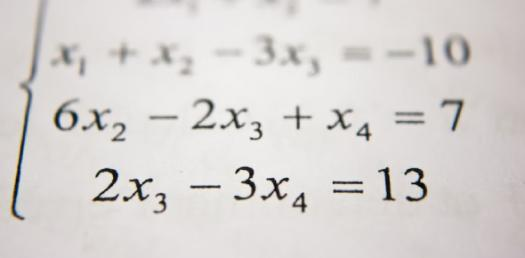# 2nd Grade Math Equation Quiz

10 Questions | Attempts: 554SettingsAre you in 2nd grade? Looking for a 2nd grade math equation quiz? We have brought one for you. In this quiz, you will come across various math questions that will include addition, subtraction, multiplication, and division. These questions will enhance your math skills, and you will get even better at calculation. So, take this quiz and also share this with your friends who are in 2nd grade. All the best to you.

• 1.
Solve this equation:3 + 7 =
• A.

9

• B.

10

• C.

13

• D.

27

• 2.
Solve this equation:87 - 46 =
• A.

37

• B.

39

• C.

40

• D.

41

• 3.
Solve this equation:97 + 39 + 29 + 10 =
• A.

111

• B.

127

• C.

165

• D.

175

• 4.
Solve this equation:167 - 62 =
• A.

105

• B.

116

• C.

136

• D.

141

• 5.
Solve this equation:50/5 =
• A.

5

• B.

10

• C.

15

• D.

20

• 6.
Solve this equation:45 x 10 =
• A.

350

• B.

400

• C.

450

• D.

550

• 7.
Solve this equation:24/6 =
• A.

2

• B.

5

• C.

3

• D.

4

• 8.
Solve this equation:7 x 13 =
• A.

91

• B.

93

• C.

100

• D.

105

• 9.
Solve this equation:165 - 55 =
• A.

75

• B.

96

• C.

105

• D.

110

• 10.
Solve this equation:67 + 76 =
• A.

143

• B.

152

• C.

162

• D.

170

## Related TopicsBack to top
×

Wait!
Here's an interesting quiz for you.